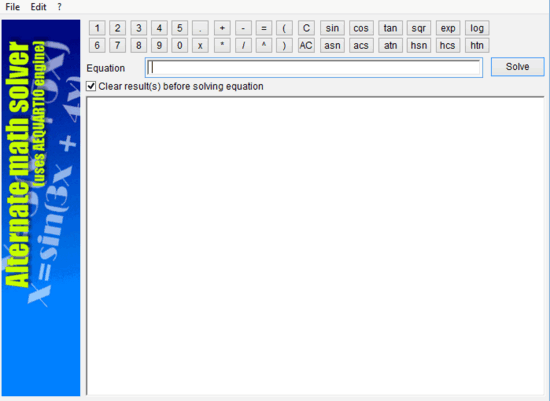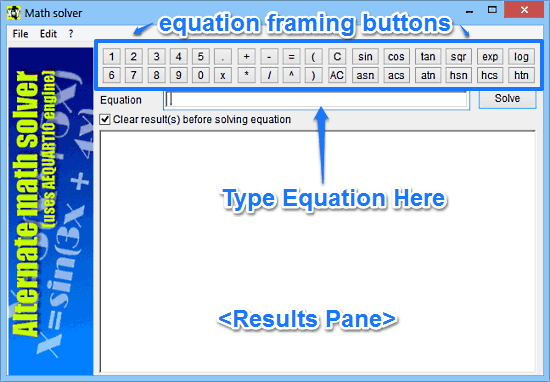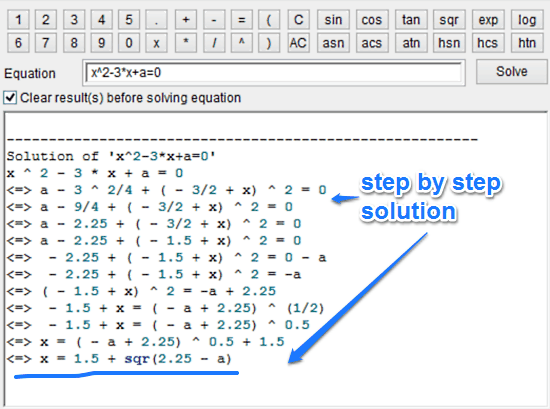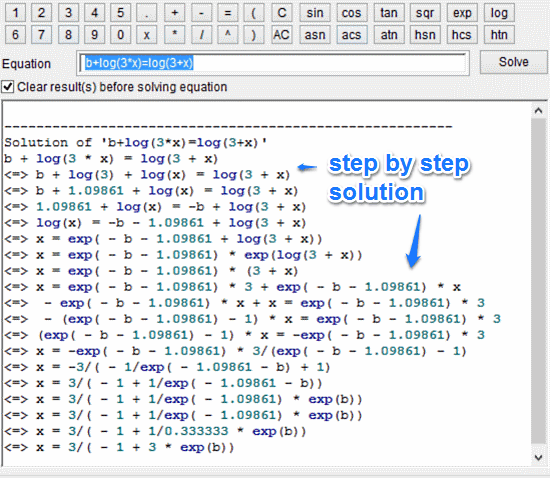Editor Ratings:
User Ratings:
[Total: 0 Average: 0]

Alternate Math Solver is a free software to solve mathematical equations. It’s one of those software applications that students will find really helpful in their studies. Simple and easy to use, Alternate Math Solver is a lightweight application that lets you solve simple (and somewhat complex!) mathematical equations. It can solve algebraic equations of different degrees, having one or more constants. Equations are always solved for the unknown variable ‘x’. This means that as long as there is a single instance of ‘x’ in the equation, the application will solve it to return an answer. Apart from standard equation solving, Alternate Math Solver also includes a bunch of built-in mathematical functions (e.g. trigonometric, exponential and logarithmic) that can be used to frame equations as well. Sounds like something that can help you with your homework? Let’s find out more!## How To Use Alternate Math Solver To Solve Mathematical Equations?

Before getting started with Alternate Math Solver, the first step is to install the application. At just about 680 KB, it’s a pretty featherweight application and as such, installation is a breeze. All you have to do is download the installer from the homepage, install it, and you’re all set. Once installed, Alternate Math Solver should fire up automatically. Here’s how the user interface looks like:As illustrated by the above screenshot, Alternate Math Solver has a pretty standard and straightforward interface. Primarily, it’s comprised of a Results Pane, which is just a rectangular area where the step by step solutions of your entered equations appear. Apart from that, there’s an Equation field, where your entered equation appears. The top consists of a bunch of virtual buttons, having numbers, mathematical operators and different functions. These buttons are used to frame the equations to be solved. Pretty simple stuff if you ask me!

Using Alternate Math Solver to find quick solutions to your mathematical equations is really easy. Essentially, all you have to do is frame the equation using the buttons, and hit the Solve button. Here’s a little step by step tutorial anyway, illustrating how to use Alternate Math Solver via a few sample equations:

Step 1: Using the buttons provided above, frame the mathematical equation that you’d like to solve. Note that the equation must contain an unknown variable ‘x’, as the equation will be solved for this value. Moreover, you need to properly format the equation (e.g. include both LHS and RHS, for it’s an equation). As our first example, let’s use the equation: x^2-3*x+a=0. This essentially is a quadratic equation (of degree 2), translating to x squared minus 3 times 2 plus a equals to 0. Once you hit the Solve button, its result is instantly calculated and shown in the results window:As illustrated by the above screenshot, the Alternate Math Solver gives detailed step by step solution of the equation. Let’s try out another equation, having some mathematical functions.

Step 2: As the next example, take the equation: b+log(3*x)=log(3+x) This equation involves logarithmic functions, and is read as b plus log of 3 times x equals to log of 3 plus x. As in the previous example, all you have to do is hit the Solve button. Here’s what the solution looks like:Pretty cool, right? That’s all there’s to it! All you have to do is frame your equations using the correct syntax, and hit the Solve button. If you want, you can even save the result as a text file, using the File > Save Results option.

## Conclusion:

Alternate Math Solver is a really useful free software to solve mathematical equations. It’s simple, easy to use, and has quite a handy selection of built-in mathematical functions. Features like speedy calculation, detailed step by step solutions and the ability to export the results really make it a powerful application, and a must have for any student. Do give this a try, you’ll love it.

Get Alternate Math Solver Here.